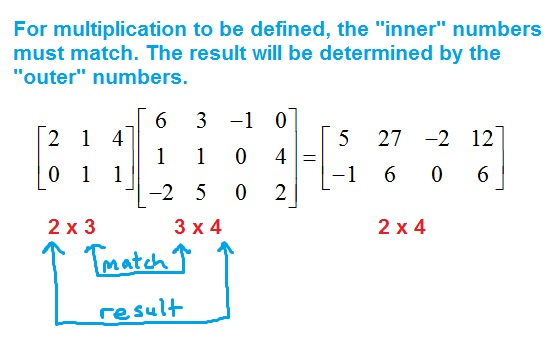# Multiplying matrices

While adding or subtracting matrices is relatively straightforward, multiplying matrices is very different from most mathematical operations you have learned beforehand. Here, we will review a nice way to multiply two matrices and some important properties associated with it. You will also learn how to tell when the multiplication is undefined.

## Multiplying two matrices: “rows hit columns”

To understand the general pattern of multiplying two matrices, think “rows hit columns and fill up rows”. Consider the following example.

The first row “hits” the first column, giving us the first entry of the product. Notice that since this is the product of two 2 x 2 matrices (number of rows and columns), the result will also be a 2 x 2 matrix. We will look at how the size of the matrix affects this later in the article.Now, the first row “hits” the second column, filling up the row of the product.Having run out of columns to “hit”, we now work with the second row.There is one last entry to calculate. The second row will now “hit” the second column.Finally, we just need to complete the arithmetic to get our final answer.### An animation of this process

You can see an animation of this process here. There is no sound – so don’t worry about finding your headphones!

We will see a couple more examples shortly, but first, we need to discuss how the size of a matrix affects the result when multiplying. In fact, there are cases where due to the size of the matrix, the multiplication is undefined.

## Matrix multiplication is not always defined

When multiplying matrices, the size of the two matrices involved determines whether or not the product will be defined. You can also use the sizes to determine the result of multiplying the two matrices. Recall that the size of a matrix is the number of rows by the number of columns. The matrices above were 2 x 2 since they each had 2 rows and 2 columns.As you can see, the sizes of the matrices do not have to be the same, you just need the middle two numbers to match when you write the sizes side by side. Otherwise, the product is undefined.Think about this: if a matrix A is 3 x 4, for example, then the product of A and itself would not be defined, as the inner numbers would not match. This is just one example of how matrix multiplication does not behave in the way you might expect.

## Matrix multiplication is not commutative

You know from grade school that the product (2)(3) = (3)(2). It doesn’t matter which order you multiply the numbers in, the result is the same. This does not work in general for matrices. Only in special cases can you say that AB = BA. So, in general, you should assume that they are not equal. It can even be the case that AB is defined, while BA is not defined!Even if the product is defined, again, it is unlikely the results will be the same for AB and BA.## Examples of multiplying matrices

Now that we have seen some of the important properties of matrix multiplication, let’s work through a couple of examples.

### Example

Find the product AB where:
$$A = \left[\begin{array}{cc} -5 & 3\\ -4 & -1\\ \end{array}\right]$$
and
$$B = \left[\begin{array}{cc} 1 & -1\\ 2 & 6\\ \end{array}\right]$$

### Solution

Remember that rows hit columns, and fill up rows. Here, the matrices are each 2 x 2 and so the result will be a 2 x 2 matrix.

\begin{align} AB &= \left[\begin{array}{cc} -5 & 3\\ -4 & -1\\ \end{array}\right] \left[\begin{array}{cc} 1 & -1\\ 2 & 6\\ \end{array}\right]\\ &= \left[\begin{array}{cc} -5(1) + 3(2) & -5(-1) + 3(6)\\ -4(1) +(-1)(2) & (-4)(-1)+(-1)(6)\\ \end{array}\right]\\ &= \boxed{\left[\begin{array}{cc} 1 & 23\\ -6 & -2\\ \end{array}\right]}\end{align}

### Example

Find the product AB where:

$$A = \left[\begin{array}{cccc} -2 & -1 & 0 & 0 \\ 1 & 2 & 1 & 1\\ \end{array}\right]$$
and
$$B = \left[\begin{array}{cccc} 3 & 1 & 1 & 2 \\ -1 & -1 & 0 & 1\\ \end{array}\right]$$

### Solution

Here, we have a 2 x 4 matrix multiplied by a 2 x 4 matrix. The inner numbers of these sizes do not match, therefore:

$$\boxed{AB \text{ is undefined}}$$

### Example

Find the product AB where:
$$A = \left[\begin{array}{cc} 1 & 2\\ -2 & 0\\ 3 & 1\\\end{array}\right]$$

and
$$B = \left[\begin{array}{cc} 4 & 0\\ 0 & 1\\ \end{array}\right]$$

### Solution

This is the product of a 3 x 2 matrix and a 2 x 2 matrix. The inner numbers match, so the product is defined. The result will be a 3 x 2 matrix.

\begin{align} AB &= \left[\begin{array}{cc} 1 & 2\\ -2 & 0\\ 3 & 1\\\end{array}\right]\left[\begin{array}{cc} 4 & 0\\ 0 & 1\\ \end{array}\right]\\ &= \left[\begin{array}{cc} 1(4) + 2(0) & 1(0) + 2(1)\\ -2(4) + 0(0) & -2(0) + 0(1)\\ 3(4) + 1(0) & 3(0) + 1(1)\\\end{array}\right]\\ &= \boxed{\left[\begin{array}{cc} 4 & 2\\ -8 & 0\\ 12 & 1\\\end{array}\right]}\end{align}